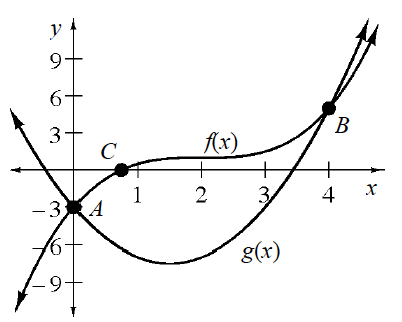### Home > A2C > Chapter 5 > Lesson 5.1.2 > Problem5-26

5-26.

Consider the graphs of $f\left(x\right)=\frac{1}{2}(x−2)^3+1$ and $g(x)=2x^2−6x−3$ at right.

1. Write an equation that you could solve using points $A$ and $B$. What are the solutions to your equation? Substitute them into your equation to show that they work.

Set $f(x)$ and $g(x)$ equal to each other: $\frac{1}{2}(\textit{x}-2)^{3}+1=2\textit{x}^{2}-6\textit{x}-3$

2. Are there any solutions to the equation in part (a) that do not appear on the graph? Explain.

Solve the equation for $x$.

$\frac{1}{2}\left(\textit{x}^{3}-6\textit{x}^{2}+12\textit{x}-8\right)-2\textit{x}^{2}+6\textit{x}+4=0$

$\frac{1}{2}\textit{x}^{3}-5\textit{x}^{2}+12\textit{x}=0$

$\textit{x}\left(\frac{1}{2}\textit{x}-3\right)(\textit{x}-4)=0$

3. Write an equation that you could solve using point $C$. What does the solution to your equation appear to be? Again, substitute your solution into the equation. How close was your estimate?

Set $f(x)$ equal to $0$.

4. What are the domains and ranges of $f(x)$ and $g(x)$?

Domain of $f(x)$: All real numbers

Range of $f(x)$: All real numbers

Domain of $g(x)$: All real numbers

Range of $g(x):y\ge−7.5$